Courses

# Test: Number System- 1

## 15 Questions MCQ Test General Aptitude for GATE 2020 | Test: Number System- 1

Description
This mock test of Test: Number System- 1 for Quant helps you for every Quant entrance exam. This contains 15 Multiple Choice Questions for Quant Test: Number System- 1 (mcq) to study with solutions a complete question bank. The solved questions answers in this Test: Number System- 1 quiz give you a good mix of easy questions and tough questions. Quant students definitely take this Test: Number System- 1 exercise for a better result in the exam. You can find other Test: Number System- 1 extra questions, long questions & short questions for Quant on EduRev as well by searching above.
QUESTION: 1

### How many factors of 25 * 36 * 52 are perfect squares?

Solution:

Any factor of this number should be of the form 2a * 3b * 5c.
For the factor to be a perfect square a,b,c have to be even.
a can take values 0, 2, 4.
b can take values 0, 2, 4, 6.
and c can take values 0, 2.

Total number of perfect squares = 3 * 4 * 2 = 24

QUESTION: 2

### The sum of the first 100 natural numbers, 1 to 100 is divisible by

Solution:

The sum of the first 100 natural numbers is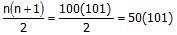.

101 is an odd number and 50 is divisible by 2.

Hence, 50*101 will be divisible by 2.

QUESTION: 3

### Convert the following binary number to decimal (010111)2?

Solution:

To do this, at first translate it to decimal here so:
(010111)2 = 0∙25+1∙24+0∙23+1∙22+1∙21+1∙20 = 0+16+0+4+2+1 = 2310

Result of converting:
(010111)2 = 2310

QUESTION: 4

Find the greatest five-digit number that is exactly divisible by 7, 10, 15, 21 and 28.

Solution:

Step 1: Prime factorize all the divisors to find the least number of tests to be done.
The number should be divisible by 10 (2, 5), 15 (3, 5), 21 (3, 7), and 28 (22, 7).
Hence, it is enough to check whether the number is divisibile by 3, 22, 5 and 7. i.e., for 3, 4, 7, and 7.

Tests for divisibility with number 3, 4, 5, and 7?

Test of divisibility by 3: Sum of the digits will be divisible by 3 if a number is divisible by 3. For e.g., sum of the digits of 345 is 3 + 4 + 5 = 12. 12 is divisible by 3. So, 345 is also divisible by 3.
Test of divisibility by 4: The righmost two digits viz., the units and tens digits will be divisible by 4 if a number is divisible by 4. For e.g, 1232 is divisible by 4 because 32 is divisible by 4.
Test of divisibility by 5: The units digit is either 5 or 0.
Test of divisibility by 7: For up to 5 digit numbers, the quickest way is to actually divide the number by 7 and check out.

Step 2: Go from answer choices

Choice 1: 99840 : The number is divisible by 3, 4, and 5. But the number is not divisible by 7.

Choice 2: 99900 : The number is divisible by 3, 4, and 5. But the number is not divisible by 7.

Choice 3: 99960 : The number is divisible by all 3, 4, 5, and 7.
Choice 4: 99990 : The number is not divisible by  4, and 7.

QUESTION: 5

N! is having 37 zeros at its end. How many values of N is/are possible?

Solution:

The number of zero is determined by the nos. of 5's & 2's,whichever is less. (since 5 x 2 =10).

In most of the cases number of 5's is less than that of 2's.

Now in the question,

A/q, N/5 + N/5+ N/5+ N/5= 37

⇒This gives the value of N=150,151,152,153,154

⇒ 5 values.

QUESTION: 6

P is a natural number. 2P has 28 divisors and 3P has 30 divisors. How many divisors of 6P will be there?

Solution:

If 2P has 28 divisors then 2P = (26)(33)

If 3P has 30 divisors then 3P = (25)(34)

Therefore P = (25)(33)

Then 6P = 2*3*(25)(33) = (26)(34)

6P will have (6+1)(4+1) = 35 divisors

QUESTION: 7

What is the least number of soldiers that can be drawn up in troops of 12, 15, 18 and 20 soldiers and also in form of a solid square?

Solution:

In this type of question, We need to find out the LCM of the given numbers.
LCM of 12, 15, 18 and 20;
12 = 2 x 2 x 3;
15 = 3 x 5;
18 = 2 x 3 x 3;
20 = 2 x 2 x 5;
Hence, LCM = 2 x 2 x 3 x 5 x 3
Since, the soldiers are in the form of a solid square.
Hence, LCM must be a perfect square. To make the LCM a perfect square, We have to multiply it by 5, hence, the required number of soldiers = 2 x 2 x 3 x 3 x 5 x 5 = 900.

QUESTION: 8

How many factors of 1080 are perfect squares?

Solution:

Factors of 1080 are
1, 2, 3, 4, 5, 6, 9, 10, 12, 15, 18, 20, 24, 27, 30, 36, 40, 45, 54, 60, 90, 120, 135, 180, 270, 360, 450, 540, 1080
The factors of 1080 which are perfect square are 1,4,9,36 which is 4 in total

QUESTION: 9

Rohan purchased some pens, pencils and erasers for his young brothers and sisters for the ensuing examinations. He had to buy atleast 11 pieces of each item in a manner that the number of pens purchased be more than the number of pencils, which is more than the number of erasers. He purchased a total of 38 pieces.

If the number of pencils cannot be equally divided among his 4 brothers and sisters, how many pens did he purchase?

Solution:

Different possibilities for the number of pencils = 12 or 13. Since it cannot be divided into his 4 brothers and sisters, it has to be 13. In that case, number of pens = 14

QUESTION: 10

The representation of decimal number 532.86 in the form of binary - decimal is

Solution:

Whole part of a number is obtained by dividing on the basis new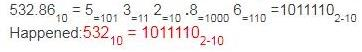QUESTION: 11

1 ’s are given 100 times, 2 ’s are given 100 times and 3’s are given 100 times. Now numbers are made by arranging these 300 digits in all possible ways. How many of these numbers will be perfect squares?

Solution:

The digital sum of any perfect square can be 1,4, 9, 7, 0 only. 100 tim es l ’s are given, 100 times 2 ’s are given and 100 times 3’s are given.

Sum of the digits: 100+200+300=600

The number would be divisible by 3, but not by 9.

Digital sum of all these digits taken all at a time will be 6. So, this will not give any perfect square.

QUESTION: 12

Find the remainder when 73 *75 *78 *57 *197 *37 is divided by 34.

Solution:

Remainder,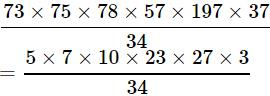[We have taken individual remainder, which means if 73 is divided by 34 individually, it will give remainder 5, 75 divided 34 gives remainder 7 and so on.]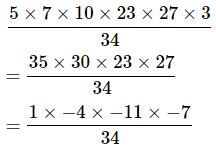[We have taken here negative as well as positive remainder at the same time. When 30 divided by 34 it will give either positive remainder 30 or negative remainder -4. We can use any one of negative or positive remainder at any time.]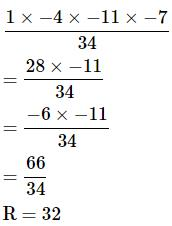QUESTION: 13

There are two integers 34041 and 32506, when divided by a three-digit integer n, leave the same remainder. What is the value of n?

Solution:

Find the difference between both 34041 and 32506.

34041 - 32506 = 1535

So N should be a factor of 1535.

Factors of 1535:-

1, 5, 307, 1535

Here, 307 is a three digit factor of 1535. So N = 307

34041 and 32506 divided by 307 leaves remainder 271.

QUESTION: 14

Find the highest power of 24 in 150!

Solution:

24 = 8 × 3. Therefore, we need to find the highest power of 8 and 3 in 150!

8 = 23⇒ highest power of 8 in 150! = [(150/2) + (150/4) + (150/8) +(150/16) + (150/32) + (150/64) +(150/128)] /3 =48

Highest power of 3 in 150! = [150/3]+[150/9]+[150/27]+[150/81] = 72

As the powers of 8 are less, powers of 24 in 150! = 48.

QUESTION: 15

Teacher said that there were 100 students in his class, 24 of whom were boys and 32 were girls. Which base system did the teacher use in this statement?

Solution:

Explanation:
We are provided with the equation (32) + (24) = (100)
Let us assume our base be 'b'
Then,we can say
32 = 3 x b1+2 x b0 = 3b+2
Similarly,
24 = 2 x b1+4 x b= 2b+4
100 = 1 x b2+0 x b+ 0 x b0 = b2

Now , according to our question,
32+24=100
(3b+2) + (2b+4) = (b2)
5b + 6 = b2
b- 5b - 6 = 0
b- 6b + b - 6 = 0
b(b-6) + 1(b-6) = 0
(b-6)(b+1) = 0
b=6,-1
Base can't be negative. Hence b=6
Therefore base assumed in the asked question must be 6